1201904 — Published On February 12, 2017 and Last Modified On June 24th, 2022

## Introduction

Ensemble modeling is a powerful way to improve the performance of your machine learning models. If you wish to be on the top of leaderboard in any machine learning competition or want to improve models you are working on – ensemble is the way to go. The meme below kind of summarizes the power of ensembling: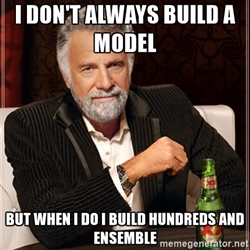Given the importance of ensemble modeling, we decided to test our community on ensemble modeling. The test included basics of ensemble modeling and its practical applications.

A total of 1411 participants registered for the skill test. If you missed taking the test, here is your opportunity for you to find out how many questions you could have answered correctly.## Overall Results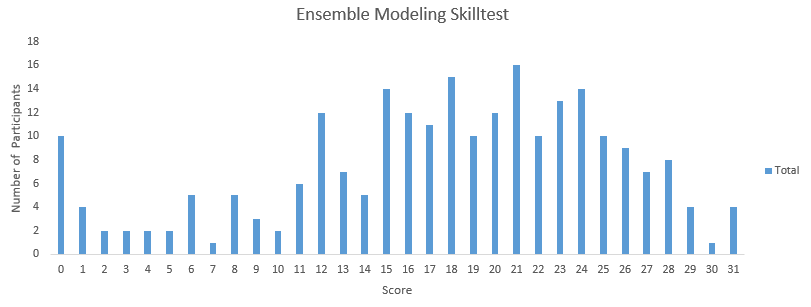You can access your performance here. More than 230 people participated in the skill test and the highest score was 31. Here are a few statistics about the distribution.

Overall distribution

Mean Score: 17.54

Median Score: 18

Mode Score: 21

5 Easy questions on Ensemble Modeling everyone should know

Powerful ‘Trick’ to choose right models in Ensemble Learning

Basics of Ensemble Learning Explained in Simple English

If you are new to ensemble learning, we have you covered – you can enrol in this free course where we cover all the main ensemble modelling techniques in a structured and comprehensive manner: Ensemble Learning and Ensemble Learning Techniques

Q1. Which of the following algorithm is not an example of an ensemble method?

A. Extra Tree Regressor
B. Random Forest
D. Decision Tree

Q2. What is true about an ensembled classifier?

1. Classifiers that are more “sure” can vote with more conviction
2. Classifiers can be more “sure” about a particular part of the space
3. Most of the times, it performs better than a single classifier

A. 1 and 2
B. 1 and 3
C. 2 and 3
D. All of the above

Q3. Which of the following option is / are correct regarding benefits of ensemble model?

1. Better performance
2. Generalized models
3. Better interpretability

A. 1 and 3
B. 2 and 3
C. 1 and 2
D. 1, 2 and 3

Q4) Which of the following can be true for selecting base learners for an ensemble?

1. Different learners can come from same algorithm with different hyper parameters
2. Different learners can come from different algorithms
3. Different learners can come from different training spaces

A. 1
B. 2
C. 1 and 3
D. 1, 2 and 3

Q5. True or False: Ensemble learning can only be applied to supervised learning methods.

A. True
B. False

Q

6. True or False: Ensembles will yield bad results when there is significant diversity among the models.

Note: All individual models have meaningful and good predictions.

A. True
B. False

Solution: (B)

An ensemble is an art of combining a diverse set of learners (individual models) together to improvise on the stability and predictive power of the model. So, creating an ensemble of diverse models is a very important factor to achieve better results.

Q7. Which of the following is / are true about weak learners used in ensemble model?

1. They have low variance and they don’t usually overfit
2. They have high bias, so they can not solve hard learning problems
3. They have high variance and they don’t usually overfit

A. 1 and 2
B. 1 and 3
C. 2 and 3
D. None of these

Solution: (A)
Weak learners are sure about particular part of a problem. So they usually don’t overfit which means that weak learners have low variance and high bias.

Q8.True or False: Ensemble of classifiers may or may not be more accurate than any of its individual model.

A. True

B. False

Solution: (A)

Usually, ensemble would improve the model, but it is not necessary. Hence, option A is correct.

Q9. If you use an ensemble of different base models, is it necessary to tune the hyper parameters of all base models to improve the ensemble performance?

A. Yes
B. No
C. can’t say

Solution: (B)

It is not necessary. Ensemble of weak learners can also yield a good model.

Q10. Generally, an ensemble method works better, if the individual base models have ____________?

Note: Suppose each individual base models have accuracy greater than 50%.

A. Less correlation among predictions
B. High correlation among predictions
C. Correlation does not have any impact on ensemble output
D. None of the above

Solution: (A)

A lower correlation among ensemble model members will increase the error-correcting capability of the model. So it is preferred to use models with low correlations when creating ensembles.

Context – Question 11

In an election, N candidates are competing against each other and people are voting for either of the candidates. Voters don’t communicate with each other while casting their votes.

Q.11 Which of the following ensemble method works similar to above-discussed election procedure?

Hint: Persons are like base models of ensemble method.

A. Bagging
B. Boosting
C. A Or B
D. None of these

Solution: (A)

In bagged ensemble, the predictions of the individual models won’t depend on each other. So option A is correct.

Q12. Suppose you are given ‘n’ predictions on test data by ‘n’ different models (M1, M2, …. Mn) respectively. Which of the following method(s) can be used to combine the predictions of these models?

Note: We are working on a regression problem

1. Median
2. Product
3. Average
4. Weighted sum
5. Minimum and Maximum
6. Generalized mean rule

A. 1, 3 and 4
B. 1,3 and 6
C. 1,3, 4 and 6
D. All of above

Solution: (D)

All of the above options are valid methods for aggregating results of different models (in case of a regression model).

Context: Question 13 -14

Suppose, you are working on a binary classification problem. And there are 3 models each with  70% accuracy.

Q13. If you want to ensemble these models using majority voting method. What will be the maximum accuracy you can get?

A. 100%
B. 78.38 %
C. 44%
D. 70

Solution: (A)

Refer below table for models M1, M2 and M3.

 Actual output M1 M2 M3 Output 1 1 0 1 1 1 1 0 1 1 1 1 0 1 1 1 0 1 1 1 1 0 1 1 1 1 0 1 1 1 1 1 1 1 1 1 1 1 0 1 1 1 1 0 1 1 1 1 0 1

Q14. If you want to ensemble these models using majority voting. What will be the minimum accuracy you can get?

A. Always greater than 70%
B. Always greater than and equal to 70%
C. It can be less than 70%
D. None of these

Solution: (C)

Refer below table for models M1, M2 and M3.

 Actual Output M1 M2 M3 Output 1 1 0 0 0 1 1 1 1 1 1 1 0 0 0 1 0 1 0 0 1 0 1 1 1 1 0 0 1 0 1 1 1 1 1 1 1 1 1 1 1 1 1 1 1 1 1 1 1 1

Q15. How can we assign the weights to output of different models in an ensemble?

1.  Use an algorithm to return the optimal weights
2. Choose the weights using cross validation
3. Give high weights to more accurate models

A. 1 and 2
B. 1 and 3
C. 2 and 3
D. All of above

Solution: (D)

All of the options are correct to decide weights of individual models in an ensemble.

Q16. Which of the following is true about averaging ensemble?

A. It can only be used in classification problem
B. It can only be used in regression problem
C. It can be used in both classification as well as regression
D. None of these

Solution: (C)

You can use average ensemble on classification as well as regression. In classification, you can apply averaging on prediction probabilities whereas in regression you can directly average the prediction of different models.

Context Question 17

Suppose you have given predictions on 5 test observations.

predictions = [0.2,0.5,0.33,0.8]

Which of the following will be the ranked average output for these predictions?

Hint: You are using min-max scaling

A. [ 0., 0.66666667, 0.33333333, 1. ]

B. [ 0.1210, 0.66666667, 0.95,0.33333333 ]

C. [ 0.1210, 0.66666667, 0.33333333, 0.95 ]

D. None of above

Solution: (A)

The following steps can be applied to get the output in options A

1. Given ranking to the predictions
2. Scale these ranking using min max scaling

You can follow this code in python to get the desired result.

```from sklearn.preprocessing import MinMaxScaler
x = np.array([0.2,0.5,0.33, 0.8])
sc = MinMaxScaler()
sc.fit_transform(np.argsort(x))```

Q18.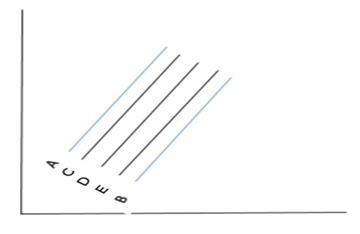In above snapshot, line A and B are the predictions for 2 models (M1, M2 respectively). Now, You want to apply an ensemble which aggregates the results of these two models using weighted averaging. Which of the following line will be more likely of the output of this ensemble if you give 0.7, 0.3 weights to models M1 and M2 respectively.

A) A
B) B
C) C
D) D
E) E

Solution: (C)

1. We want to give higher weights to better performing models
2. Inferior models can overrule the best model if collective weighted votes for inferior models is higher than best model
3. Voting is special case of weighted voting

A. 1 and 3
B. 2 and 3
C. 1 and 2
D. 1, 2 and 3
E. None of above

Solution: (D)

All of the statements are true.

Context – Question 20-21

Suppose in a classification problem, you have following probabilities for three models: M1, M2, M3 for five observations of test data set.

 M1 M2 M3 Output .70 .80 .75 .50 .64 .80 .30 .20 .35 .49 .51 .50 .60 .80 .60

Q20. Which of the following will be the predicted category for these observations if you apply probability threshold greater than or equals to 0.5 for category “1” or less than 0.5 for category “0”?

Note: You are applying the averaging method to ensemble given predictions by three models.

A.

 M1 M2 M3 Output .70 .80 .75 1 .50 .64 .80 1 .30 .20 .35 0 .49 .51 .50 0 .60 .80 .60 1

B.

 M1 M2 M3 Output .70 .80 .75 1 .50 .64 .80 1 .30 .20 .35 0 .49 .51 .50 1 .60 .80 .60 1

C.

 M1 M2 M3 Output .70 .80 .75 1 .50 .64 .80 1 .30 .20 .35 1 .49 .51 .50 0 .60 .80 .60 0

D. None of these

Solution: (B)

Take the average of predictions of each models for each observation then apply threshold 0.5 you will get B answer.

For example, in first observation of the models (M1, M2 and M3) the outputs are 0.70,0.80,0.75 if you take the average of these three you will get 0.75 which is above 0.5 that means this observation will belong to class 1.

Q21: Which of the following will be the predicted category for these observations if you apply probability threshold greater than or equals to 0.5 for category “1” or less than 0.5 for category “0”?

A.

 M1 M2 M3 Output .70 .80 .75 1 .50 .64 .80 1 .30 .20 .35 0 .49 .51 .50 0 .60 .80 .60 1

B.

 M1 M2 M3 Output .70 .80 .75 1 .50 .64 .80 1 .30 .20 .35 0 .49 .51 .50 1 .60 .80 .60 1

C.

 M1 M2 M3 Output .70 .80 .75 1 .50 .64 .80 1 .30 .20 .35 1 .49 .51 .50 0 .60 .80 .60 0

D. None of these

Solution: (B)

Take the weighted average of the predictions of each model for each observation then apply threshold 0.5 you will get B answer.

For example, in first observation of models (M1,M2 and M3) the outputs are 0.70,0.80,0.75 if you take the weighted average of these three predictions, you will get 0.745 output (0.70 * 0.4 + 0.80 *0.3 + 0.75* 0.3) which is above 0.5 that means this observation will belong to class 1.

Context: Question 22-23

Suppose in binary classification problem, you have given the following predictions of three models (M1, M2, M3) for five observations of test data set.

 M1 M2 M3 Output 1 1 0 0 1 0 0 1 1 1 0 1 1 1 1

Q22: Which of the following will be the output ensemble model if we are using majority voting method?

A.

 M1 M2 M3 Output 1 1 0 0 0 1 0 1 0 1 1 0 1 0 1 0 1 1 1 1

B.

 M1 M2 M3 Output 1 1 0 1 0 1 0 0 0 1 1 1 1 0 1 1 1 1 1 1

C.

 M1 M2 M3 Output 1 1 0 1 0 1 0 0 0 1 1 1 1 0 1 0 1 1 1 1

D. None of these

Solution: (B)

Take the majority voting for the predictions of each model for each observation.

For example for a first observation of models(M1,M2 and M3) the outputs are  1,1,0 if you take the majority voting of these three models predictions you will get 2 votes for class 1 that means this observation will belong to class 1.

Q23. When using the weighted voting method, which of the following will be the output of an ensemble model?

Hint: Count the vote of M1,  M2, and M3 as 2.5 times, 6.5 times and 3.5 times respectively.

A.

 M1 M2 M3 Output 1 1 0 0 0 1 0 1 0 1 1 0 1 0 1 0 1 1 1 1

B.

 M1 M2 M3 Output 1 1 0 1 0 1 0 0 0 1 1 1 1 0 1 1 1 1 1 1

C.

 M1 M2 M3 Ouput 1 1 0 1 0 1 0 1 0 1 1 1 1 0 1 0 1 1 1 1

D. None of these

Solution: (C)

Follow the steps in question number 20,21 and 22.

Q24. Which of the following are correct statement(s) about stacking?

1. A machine learning model is trained on predictions of multiple machine learning models
2. A Logistic regression will definitely work better in the second stage as compared to other classification methods
3. First stage models are trained on full / partial feature space of training data

A.1 and 2

B. 2 and 3

C. 1 and 3

D. All of above

Solution: (C)

1. In stacking, you train a machine learning model on predictions of multiple base models.
2. It is not necessary – we can use different algorithms for aggregating the results.
3. First stage models are trained on all the original features.

Q25. Which of the following are advantages of stacking?

• More robust model
• better prediction
• Lower time of execution

A. 1 and 2

B. 2 and 3

C. 1 and 3

D. All of the above

Solution: (A)

Option 1 and 2 are advantages of stacking whereas option 3 is not correct as staking takes higher time.

Q26: Which of the following figure represents stacking?

A.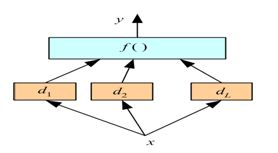B.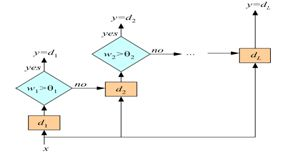C.  None of these

Solution: (A)

A is correct because it is aggregating the results of base models by applying a function f (you can say a model) on the outputs of d1, d2 and dL.

Q27. Which of the following can be one of the steps in stacking?

1. Divide the training data into k folds
2. Train k models on each k-1 folds and get the out of fold predictions for remaining one fold
3. Divide the test data set in “k” folds and get individual fold predictions by different algorithms

A. 1 and 2
B. 2 and 3
C. 1 and 3
D. All of above

Solution: (A)

The third option is not correct because we don’t create folds for test data in stacking.

Q28. Which of the following is the difference between stacking and blending?

A. Stacking has less stable CV compared to Blending
B. In Blending, you create out of fold prediction
C. Stacking is simpler than Blending
D. None of these

Solution: (D)

Only option D is correct.

Q29. Suppose you are using stacking with n different machine learning algorithms with k folds on data.

Which of the following is true about one level (m base models + 1 stacker) stacking?

Note:

• Here, we are working on binary classification problem
• All base models are trained on all features
• You are using k folds for base models

A. You will have only k features after the first stage
B. You will have only m features after the first stage
C. You will have k+m features after the first stage
D. You will have k*n features after the first stage
E. None of the above

Solution: (B)

If you have m base models in stacking. That will generate m features for second stage models.

Q30. Which of the following is true about bagging?

1. Bagging can be parallel
2. The aim of bagging is to reduce bias not variance
3. Bagging helps in reducing overfitting

A. 1 and 2
B. 2 and 3
C. 1 and 3
D. All of these

Solution: (C)

1. In bagging individual learners are not dependent on each other so they can be parallel
2-3 The bagging is suitable for high variance low bias models or you can say for complex models.

Q31.True or False: In boosting, individual base learners can be parallel.

A. True
B. False

Solution: (B)

In boosting you always try to add new models that correct previous models weaknesses. So it is sequential.

Q32. Below are the  two ensemble models:
1. E1(M1, M2, M3) and
2. E2(M4, M5, M6)

Above, Mx is the individual base models.

Which of the following are more likely to choose if following conditions for E1 and E2 are given?

E1: Individual Models accuracies are high but models are of the same type or in another term less diverse
E2: Individual Models accuracies are high but they are of different types in another term high diverse in nature

A. E1
B. E2
C. Any of E1 and E2
D. None of these

Solution: (B)

We have to select E2  because it contains diverse models. So option B is a correct option.

Q33. Suppose, you have 2000 different models with their predictions and want to ensemble predictions of best x models. Now, which of the following can be a possible method to select the best x models for an ensemble?

A. Step wise forward selection
B. Step wise backward elimination
C. Both
D. None of above

Solution: (C)

You can apply both the algorithms. In step wise forward selection, you will start with empty predictions and will add the predictions of models one at a time if they improve the accuracy of an ensemble.  In Step wise backward elimination, you will start with full set of features and remove model predictions one by one if after removing the predictions of model give an improvement in accuracy.

Q34. Suppose, you want to apply a stepwise forward selection method for choosing the best models for an ensemble model. Which of the following is the correct order of the steps?

Note: You have more than 1000 models predictions

1. Add the models predictions (or in another term take the average) one by one in the ensemble which improves the metrics in the validation set.
3. Return the ensemble from the nested set of ensembles that has maximum performance on the validation set

A. 1-2-3
B. 1-3-4
C. 2-1-3
D. None of above

Solution: (C)

Option C is correct.

Q35. True or False: Dropout is computationally expensive technique w.r.t. bagging

A. True
B. False

Solution: (B)

Because in dropout, weights are shared and the ensemble of subnetworks are trained together.

Q36.Dropout in a neural network can be considered as an ensemble technique, where multiple sub-networks are trained together by “dropping” out certain connections between neurons.

Suppose, we have a single hidden layer neural network as shown below.

How many possible combinations of subnetworks can be used for classification?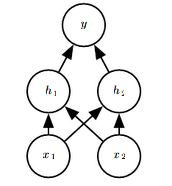How many possible combinations of subnetworks can be used for classification?

A. 1

B. 9

C. 12

D. 16

E. None of the above

Solution: (B)

There are 16 possible combinations, of which only 9 are viable. Non-viable are (6, 7, 12, 13, 14, 15, 16).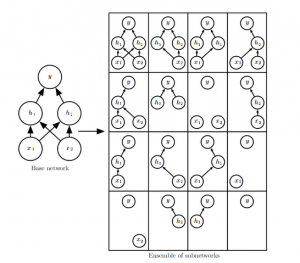Q37. How is the model capacity affected with dropout rate (where model capacity means the ability of  a neural network to approximate complex functions)?

A. Model capacity increases in increase in dropout rate

B. Model capacity decreases in increase in dropout rate

C. Model capacity is not affected on increase in dropout rate

D. None of these

Solution: (B)

The subnetworks have more number of neurons to work with when dropout rate is low. So They are more complex resulting in an increase in overall model complexity. Refer chap 11 of DL book.

Q38. Which of the following parameters can be tuned for finding good ensemble model in bagging based algorithms?

1. Max number of samples
2. Max features
3. Bootstrapping of samples
4. Bootstrapping of features

A. 1 and 3
B. 2 and 4
C. 1,2 and 3
D. 1,3 and 4
E. All of above

Solution: (E)

All of the techniques given in the options can be applied to get the good ensemble.

Q39. In machine learning, an algorithm (or learning algorithm) is said to be unstable if a small change in training data cause the large change in the learned classifiers.

True or False: Bagging of unstable classifiers is a good idea.

A. True
B. False

Solution: (A)

Refer the introduction part of this paper.

Q40. Suppose there are 25 base classifiers. Each classifier has error rates of e = 0.35.

Suppose you are using averaging as ensemble technique. What will be the probabilities that ensemble of above 25 classifiers will make a wrong prediction?

Note: All classifiers are independent of each other

A. 0.05
B. 0.06
C. 0.07
D. 0.09

Ans: B

Solution:

### End Notes

I hope you enjoyed taking the test and found the solutions helpful. The test focused on conceptual as well as practical knowledge of ensemble modeling.

If you have any doubts in the questions above, let us know through comments below. Also, If you have any suggestions or improvements you think we should make in the next skill test, you can let us know by dropping your feedback in the comments section.

## Learn, compete, hack and get hired!## 17 thoughts on "40 Questions to ask a Data Scientist on Ensemble Modeling Techniques (Skilltest Solution)"###### Saikiran says:February 13, 2017 at 8:20 am
Hi Ankit, Thanks for the solutions. It was a very good skilltest and was a very good learning experience. Just a small help: The links in the solutions for Questions 39 and 40 appear to be not working. Could you please fix that? Thanks, Sai Reply###### Sukuya says:February 13, 2017 at 8:27 am
Q37) Shouldn't the answer be B as increasing the dropout rate will reduce the model capacity. Reply###### Ankit Gupta says:February 13, 2017 at 8:50 am
Hi Saikiran, I am glad you found it useful. And also thanks for noticing, links are fixed now. Best! Ankit Gupta Reply###### Saikiran says:February 13, 2017 at 9:00 am###### Faizan Shaikh says:February 13, 2017 at 9:05 am###### Skill tests – Datafuture says:February 14, 2017 at 4:05 am
[…] You can find the questions and solutions to ensemble learning skill test here. […] Reply###### bavana princy says:February 14, 2017 at 4:54 am
This blog explains the details about changing the ways of doing that business. That is understand well and doing some different process. Provides he best output of others. Thanks for this blog Reply###### Ankit Gupta says:February 14, 2017 at 5:44 am
Hi Bavana, Thanks for your positive feedback :) Best! Ankit Gupta Reply###### Janpreet Singh says:February 14, 2017 at 2:59 pm
In Q.36) the solution takes in the scenario where it drops the input itself, I just wanted to know if that's legit? Does it brings more of a bagging essence in our model as we made our model based only on certain inputs? If you could provide the related literature also. Thank you! Regards. :) Reply###### Swapnil Rai says:February 15, 2017 at 6:31 am
I am not able to foollow solution to q13,14...can someone explain the logic...thanks! Reply###### Faizan Shaikh says:February 15, 2017 at 6:57 am
Hey Janpreet, Dropout is mostly similar to bagging in sense of how it works. So you are right on that point. For dropping input units, you can refer the paper where dropout was first introduced (http://www.jmlr.org/papers/volume15/srivastava14a.old/source/srivastava14a.pdf). It says "(dropout) provides a way of approximately combining exponentially many different neural network architectures efficiently. The term “dropout” refers to dropping out units (hidden and visible) in a neural network.". This mentions visible units (aka inputs) can be dropped out too. Hope this clears your doubt. Reply###### Ankit Gupta says:February 15, 2017 at 6:59 am
Hi Swapnil, In question number 13th For all observations, actual class is 1. Now, M1, M2 and M3 are three models each are 70% accurate (out of 10 observations 7 are same as actual output). According to the question you are using weighted majority votes on these models, means if any 2 models predictions are same then the output will belong to that class. For example, outputs of each model for first observation are 1,0,1 and in that class 1 in a majority. So the final output will belong to class 1. You can apply same procedure for all observations in questions 13, 14. Hope this answer will clarify your doubts Best! Ankit Gupta Reply###### Sukuya says:February 15, 2017 at 12:33 pm
I was able to come up with the necessary expression for question 40, but is there any way to solve it without resorting to write a program. ? Reply###### rinda jones says:March 20, 2017 at 1:11 am
Below is my answers to Q.17 in R : p <- c(0.2,0.5,0.33,0.8) mm <- function(x){(x-min(x))/(max(x)-min(x))} mm(p) ##  0.0000000 0.5000000 0.2166667 1.0000000 library(scales) rescale(p) ##  0.0000000 0.5000000 0.2166667 1.0000000 Am I wrong? If so, please tell me why? Thanks. Reply###### Ankit Gupta says:March 20, 2017 at 5:39 am
Hi Rinda, First you have to apply ranking on the predictions then min-max Scaling. Best! Ankit Gupta Reply###### rinda jones says:March 21, 2017 at 10:45 pm
Oh, I've got it mm(rank(p)) ##  0.0000000 0.6666667 0.3333333 1.0000000 Thank you, Ankit. Reply